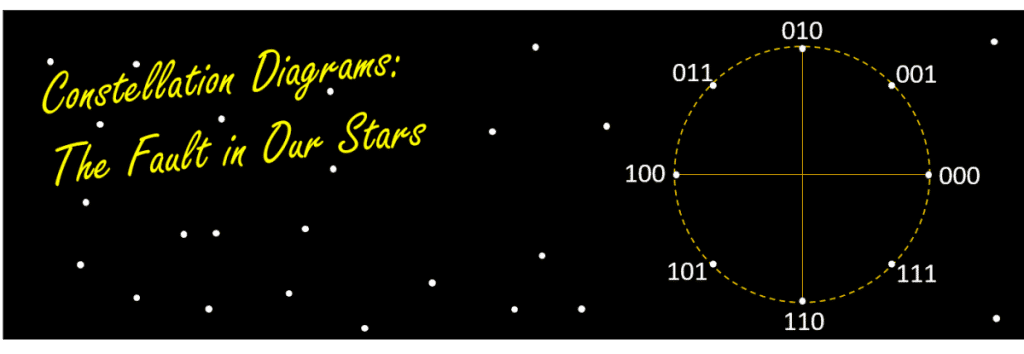Constellation diagrams are 2D graphical representations of digitally modulated signals.  They are used for the display and analysis of digitally modulated waveforms, and provide valuable insight into the performance of a digital communication system.

Digitally modulated signals encode information within a sinusoidal carrier wave by mixing it with a discretized message signal, therefore modifying the amplitude and/or phase angle of the carrier’s waveform. The resulting modulated signal has a finite combination of amplitude and phase values, which correspond to a finite number of symbols which can be used to convey information.  These symbols are often represented in polar format on the complex plane in what is known as a “constellation diagram” with each possible symbol mapped as a “constellation point”.  The location of each point on the diagram is determined by the amplitude and phase angle associated with its corresponding symbol.

The exact number of symbols (i.e. constellation points) in a modulation scheme’s digitized “alphabet” is dependent upon the type of modulation scheme used.  The amount of information that can be transmitted with a single symbol increases with the size of a modulation scheme’s alphabet.  For example, binary phase-shift keying (BPSK) has just two possible symbols and can therefore transmit just one bit per symbol: 0 or 1.  On the other hand, quadrature phase-shift keying (QPSK) has four possible symbols and can therefore transmit two bits per symbol: 00, 01, 10, or 11.  Other modulation schemes, such as quadrature amplitude modulation (QAM) can have, in practice, as many as 256 possible symbols.  As a result, each modulation scheme has its own unique constellation diagram (Fig. 1).